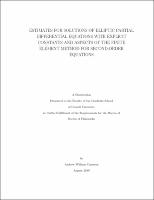## Estimates For Solutions Of Elliptic Partial Differential Equations With Explicit Constants And Aspects Of The Finite Element Method For Second-Order Equations##### Abstract
The classic Lp -based estimates for solutions of elliptic partial differential equations satisfying general boundary conditions were obtained by Agmon, Douglis, and Nirenberg in 1959. In Chapter 2, we rework these estimates to make their dependence on p explicit. It has long been believed that p enters these estimates as a single multiplicative factor of (p [-] 1)[-]1 for p close to 1 and p for p large. This is verified for second-order equations with boundary conditions of order at most one. Poorer results are obtained for more general problems. Local estimates for solutions of homogeneous equations satisfying homogeneous boundary conditions are also established. These are shown to be independent of p. Now consider the finite element approximation of a solution of a second-order elliptic partial differential equation. A typical finite element space that we consider is the Lagrange space of continuous functions which are piecewise polynomials on the elements of an unstructured but quasiuniform triangulation of the domain. As proved by Schatz in 1998, the finite element error is localised in the sense 1 that its L[INFINITY] and W[INFINITY] norms in a region depend most strongly on the behaviour of the true solution at points closest to that region. In Chapter 3, we show that the pattern in the positive norm error estimates continues into the L[INFINITY] -based negative norms. In particular, the error is localised in the negative norms in the same sense that it is in the positive norms. 1 A class of a posteriori W[INFINITY] estimators for the finite element error was inves- tigated by Hoffman, Schatz, Wahlbin, and Wittum in 2001 for the homogeneous Neumann problem. In Chapter 4, we obtain analogous results for an analogous class of L[INFINITY] estimators. Conditions are given under which these are asymptotically equivalent and asymptotically exact. One specific concrete example is provided. In the finite element approximation for the homogeneous Dirichlet problem, the computational domain does not typically match the domain on which the original problem is posed. In Chapter 5, we investigate this issue in conjunction with numerical integration. We find that superparametric elements preserve the 1998 1 weighted L[INFINITY] and W[INFINITY] error estimates of Schatz.
2011-01-31
##### Keywords
partial differential equations; finite element method; numerical analysis
##### Committee Chair
Schatz, Alfred Harry
##### Committee Member
Van Loan, Charles Francis
Wahlbin, Lars Bertil
Mathematics
##### Degree Name
Ph. D., Mathematics
##### Degree Level
Doctor of Philosophy
##### Types
dissertation or thesis